# Translations of Encyclopedia about Mathematics

## Rational Numbers: Fractions, Decimal Numbers

The set of rational numbers includes all numbers which are quotients of two whole numbers, such as with the fraction: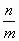, where n and m are whole numbers and where m does not equal to 0. The relating set is referred to as Q.

If we would want to divide 10 apples among 5 people, the answer is simple: each person receives two apples and the arithmetical function is 10/5.is referred to as : numerator/denominator.

We read the fraction as "ten fifths". The numerator above the line gives the number of divided units, where the denominator informs into how many parts the numerator is to be divided. In this case, it is easier to write 2 instead of.

However, if we had 5 apples and 10 people to divide it among, we could not express the answer using natural numbers because such numbers must be whole. Therefore, for this, we would need fractions or decimal numbers (numbers with a decimal point in it).

In this second case, the fraction would be, which we would read as ten fifths and the result of which would be ½ (one half), expressed as a fraction, or 0.5 if expressed as a decimal number.

Some fractions have a set name.

Basic fractions always have a numerator of 1, such as ½, ¼, 1/8, 1/16,....

With true fractions, the numerator is always smaller than the denominator, such as 2/3, 1/7, 3/100, …

If the denominator is larger than the numerator, we speak of false fractions, such as 3/2, 9/2, 100/10, and so on. In this case, the fraction may be expressed as a whole number or a "mixed" number (10/5 = 2 or 3/2 = 1 ½).

The conversion of mixed numbers to fractions is accomplished in the following manner: we multiply the number before the fraction by the fraction’s denominator and add to it the numerator. The result is a fraction numerator which is larger than the denominator.

For example: 1 ¾ = 7/4

(1*4+3 = 7)

Fractions with the same denominator are referred to as being the "same" and can be easily compared, such as: 2/5, 1/5, 9/5 etc.

If fractions have differing denominators, they are not easily comparable, such as: 2/3, 5/8, 1/6, etc.

If there is a pair of fractions in which the values of their numerators and denominators are the same but reversed, we call them reciprocal fractions, such as 2/3 and 3/2, 4/5 and 5/4, 12/8 and 8/12, etc.

Fractions can be expanded or shortened and are used for solving larger numerical functions or to simplify other fractions. The value of a fraction does not change if it is expanded or shortened.

When expanding a fraction, it numerator and denominator are multiplied by the same number: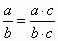When shortening, then divided by the same number:However, c can never be the number 0. The denominator cannot be the number 0 either.

Taking the fraction 24/6 as an example, we first try to see whether 24 can be divided by 6. Since it can, we arrive at:In this case, our fraction turned out to equal a whole number. However, the fraction 6/18 is easier to understand as 2/6 or 1/3.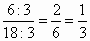Therefore, the expanding or shortening of fractions is only done whenever we want to convert several fractions to the same denominator. If, for example, we have the fractions 1/6, 1/12 and 4/3, we are not able to convert the first two fractions into thirds, otherwise their numerators would be smaller than 1. This is why we rather convert all of the fractions into expressions of twelfths.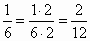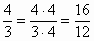Fractions can also be written as decimal numbers (those having a decimal point), where decimal points can, conversely, be written as fractions.

Therefore, 4/3 = 1,3333333333…

Or 0,166666666….= 1/6

The decimal expression of a rational number can either be final or periodic. A periodic decimal number has an unlimited number of digits following the decimal point, although a set of digits may repeat themselves following a certain point. For example, the fraction 10/4 can be written as 2.5 or 4/3 as the periodic decimal number 1.3333333……

Periodic decimal numbers are often written with a line above the repeating sequence of numbers, such as:

2,457457457…is expressed as 2,457 or 2,333…. as 2,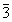.

It is often sufficient to express periodic decimal numbers only approximately or in short. So that we can express the shortened form of a number accurately, the last digit is rounded upwards or downwards, always to the nearest higher or lower number. If the last number after the last chosen point larger or equal to 5, then we round the last chosen digit upwards. If however the digit following the last chosen digit equals 5 but only because we rounded that number upwards, then the chosen digit is rounded downwards.

If we say that a number is expressed to the fourth decimal, it means that the fifth decimal point is rounded. This would mean that the number 1,33333333 would be expressed as 1,33330 and 0,166666666666 as 1,66667.

Translating Polish Portuguese Translations Portuguese Polish Translating German Swedish Translations Swedish German Translating TurkishEnter your search terms Submit search form